## #StackBounty: #python #numpy #optimization #cvxpy Inconsistency in solutions using CVXPY

### Bounty: 50

Please, consider the following optimisation problem. Specifically, `x` and `b` are `(1,n)` vectors, `C` is `(n,n)` symmetric matrix, `k` is an arbitrary constant and `i` is a `(1,n)` vector of ones.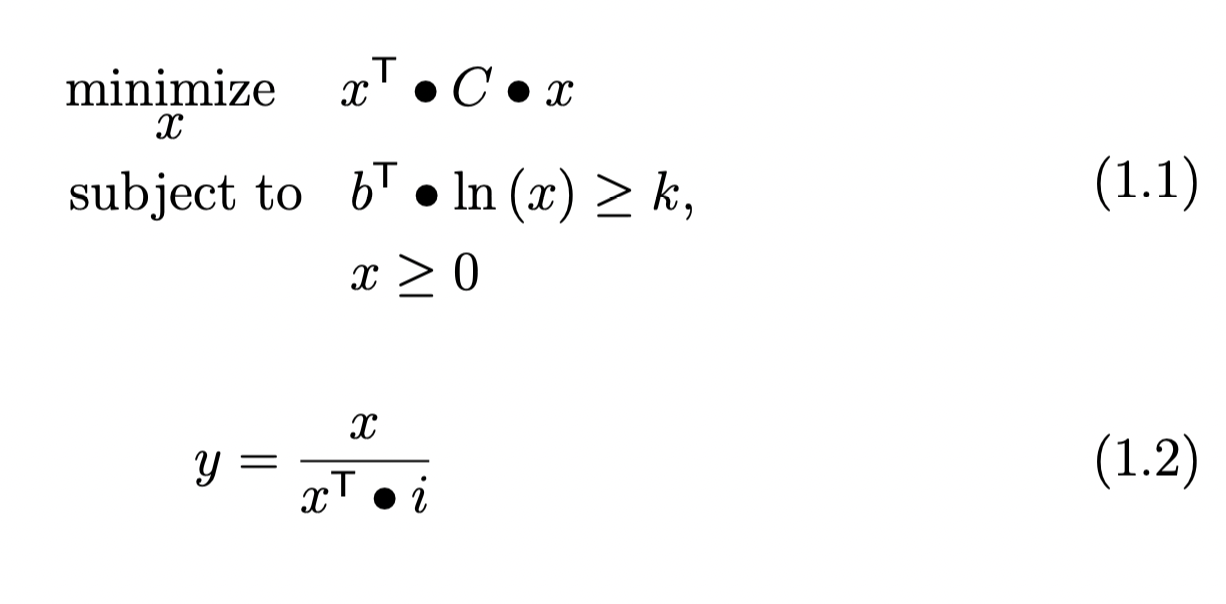Please, also consider the following equivalent optimisation problem. In such case, `k` is determined during the optimisation process so there is no need to scale the values in `x` to obtain the solution `y`.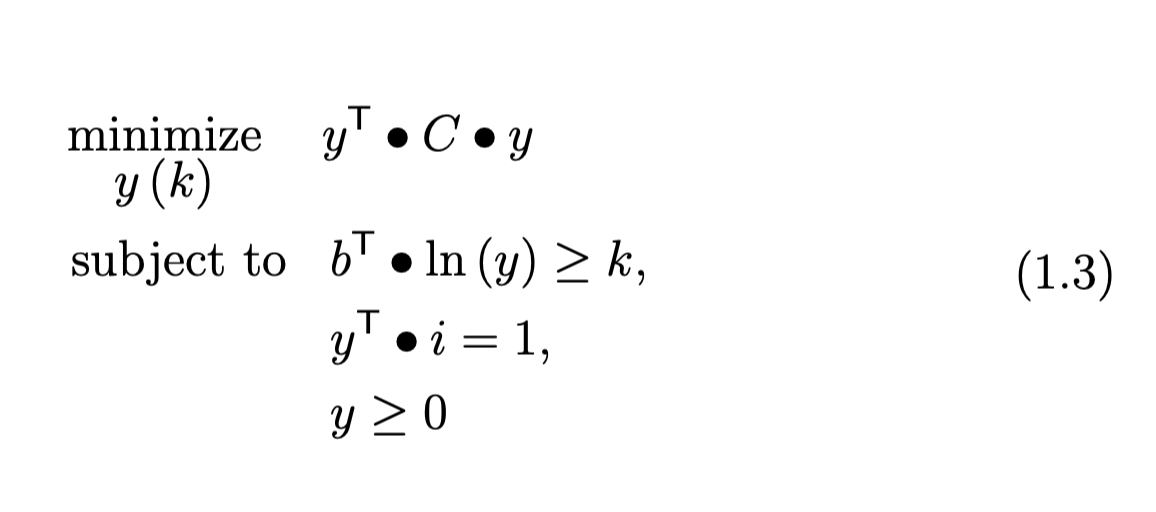Please, also consider the following code for solving both the problems with `cvxpy`.

``````import cvxpy as cp
import numpy as np

def problem_1(C):
n, t = np.shape(C)

x = cp.Variable(n)
b = np.array([1 / n] * n)

constraints = [b.T @ cp.log(x)>=0.5, w >= 0]
cp.Problem(cp.Minimize(obj), constraints).solve()

return (x.value / (np.ones(n).T @ x.value))

def problem_2(C):
n, t = np.shape(C)

y = cp.Variable(n)
k = cp.Variable()
b = np.array([1 / n] * n)

constraints = [b.T @ cp.log(y)>=k, np.ones(n)@y.T==1, y >= 0]
cp.Problem(cp.Minimize(obj), constraints).solve()

return y.value
``````

While the first function do provide me with the correct solution for a sample set of data I am using, the second does not. Specifically, values in `y` differ heavily while employing the second function with some of them being equal to zero (which cannot be since all values in `b` are positive and greater than zero). I am wondering wether or not the second function minimise also `k`. Its value should not be minimised on the contrary it should just be determined during the optimisation problem as the one that leads to the solution that minimise the objective function.

UPDATE_1

I just found that the solution that I obtain with the second formulation of the problem is equal to the one derived with the following equations and function. It appears that the constraint with the logarithmic barrier and the `k` variable is ignored.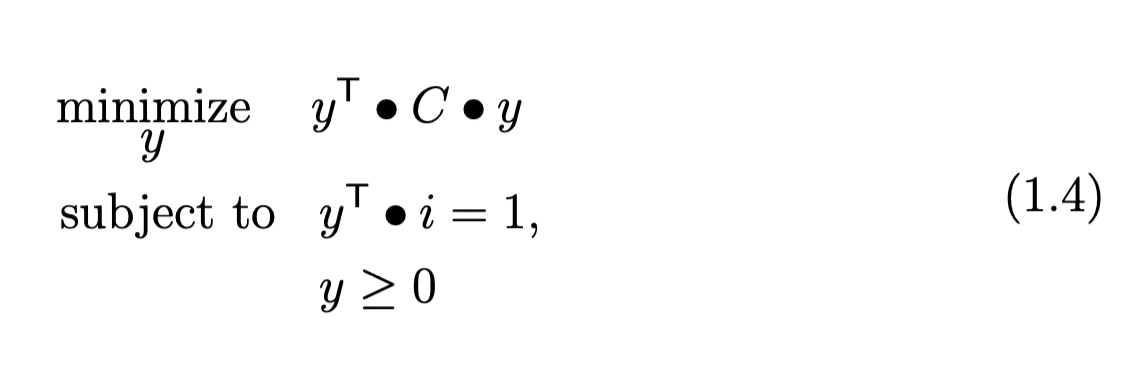``````def problem_3(C):
n, t = np.shape(C)

y = cp.Variable(n)
k = cp.Variable()
b = np.array([1 / n] * n)

constraints = np.ones(n)@y.T==1, y >= 0]
cp.Problem(cp.Minimize(obj), constraints).solve()

return y.value
``````

UPDATE_2

Here is the link to a sample input `C`https://www.dropbox.com/s/kaa7voufzk5k9qt/matrix_.csv?dl=0. In such case the correct output for both `problem_1` and `problem_2` is approximately equal to `[0.0659 0.068 0.0371 0.1188 0.1647 0.3387 0.1315 0.0311 0.0441]` since they are equivalent by definition. I am able to obtain the the correct output by solving only `problem_1`. Solving `problem_2` leads to `[0.0227 0. 0. 0.3095 0.3392 0.3286 0. 0. 0. ]` which is wrong since it happens to be the correct output for `problem_3`.

Get this bounty!!!

## #StackBounty: #optimization #computational-geometry #scheduling #intervals Finding a rainbow independent set in a circle

### Bounty: 50

Inside the interval $$[0,1]$$, there are $$n^2$$ intervals of $$n$$ different colors: $$n$$ intervals of each color. The intervals of each color are pairwise-disjoint. A rainbow independent set is a set of $$n$$ pairwise-disjoint intervals, one of each color.

A rainbow independent set always exists and can be found easily: take an interval whose rightmost endpoint is leftmost (nearest to the $$0$$). Remove all intervals of the same color and all overlapping intervals of other colors. Note that at most one interval of any other color is removed, so now we have $$n-1$$ colors with $$n-1$$ intervals of each color, and can proceed recursively.

But now, suppose that the $$n^2$$ intervals are located inside a circle (that is, an interval with both endpoints identified). Here, a rainbow independent set might not exist even for $$n=2$$, for example, if the red intervals are $$(0,0.5)$$ and $$(0.5,1)$$ and the blue intervals are $$(0.25,0.75)$$ and $$(0.75,0.25)$$.

Is there a polynomial-time algorithm to decide whether a rainbow independent set exists?

NOTE: finding a rainbow-independent-set in a general graph is NP-hard, but this is a special case.

Get this bounty!!!

## #StackBounty: #algorithms #optimization #approximation #heuristics #packing What is the approximation ratio of this bin-backing algorit…

### Bounty: 50

Consider the following algorithm for bin packing:

1. Initially, sort the items by their size.
2. Put the largest item in a new bin.
3. Fill the bin with small items in ascending order of size, up to the largest item that fits.
4. Close the bin. If some items remain, go back to step 1.

There is a very similar algorithm for the dual problem of bin covering, and its approximation ratio for that problem is 3/2. However, I did not find this algorithm discussed in the context of bin packing.

Is anything known about its approximation ratio – how close it is to the optimal solution?

Get this bounty!!!

## #StackBounty: #optimization #approximation #heuristics #packing What is the approximation ratio of this bin-backing algorithm?

### Bounty: 50

Consider the following algorithm for bin packing:

1. Initially, sort the items by their size.
2. Put the largest item in a new bin.
3. Fill the bin with small items in ascending order of size, up to the largest item that fits.
4. Close the bin. If some items remain, go back to step 1.

There is a very similar algorithm for the dual problem of bin covering, and its approximation ratio for that problem is 3/2. However, I did not find this algorithm discussed in the context of bin packing.

Is anything known about its approximation ratio – how close it is to the optimal solution?

Get this bounty!!!

## #StackBounty: #graphs #optimization #scheduling #loops Is the RecMII really important?

### Bounty: 100

Recently I’ve asked a question on SO about dependence graph and modulo scheduling, because I’m not able to get the distance of some loop carried dependency and I needed some help.

I’m building a dependence graph so that I can implement the modulo scheduler of this paper and aside for some problem that blocks me I have a question about the importance of some parameters.

In particular about the recurrence-constrained MII (RecMII).
I noticed that in some case you probably can’t get the loop carried distance of some dependency (for example `a[i]=a[f(a[i-1])] +a[i*i-2*i-1]`), so I’m asking:

• Is the RecMII really important in the modulo scheduling algorithm? If yes how much? (It just seems a way to reduce the try needed to find a valid II)
• What happens if I neglect it? Just some increase execution time of
the main loop that try to find the II or I also decrease the quality
of my scheduler?
• Can the Modulo scheduler be used only on the data dependency graph? (I’m asking this because it’s really easy to build such graph, you only need to go through the def-use chain)

Get this bounty!!!

## #StackBounty: #java #algorithm #optimization #binary Constant time for multiplication in Galois Field GF(4)

### Bounty: 50

Tl;dr: Is there a way to improve the code below in any way (including multithreading) as the code will run hundreds of billions of times?

To objective is to find a constant time algorithm (without a for loop) for performing multiplication in Galois Field GF(4). I am not sure if this is even possible but it is worth a try.

Some background: multiplication in GF(2) or base 2 is the equivalent of anding the two values being multiplied. This is because:

a b a × b = a ∧ b
0 0 0
0 1 0
1 0 0
1 1 1

For example:

``````10101011010100 × 10011000101101 =

10101011010100
10011000101101 ∧
--------------
10001000000100
``````

When it comes to GF(4), there are four different symbols that can be used: 0, 1, 2 and 3. It is not the same as performing multiplication in base 4 because some digits don’t give an expected result when multiplied by other digits. They are bolded in the table below:

a b a × b
0 0 0
0 1 0
0 2 0
0 3 0
1 0 0
1 1 1
1 2 2
1 3 3
2 0 0
2 1 2
2 2 3
2 3 1
3 0 0
3 1 3
3 2 1
3 3 2

A more compact form of the above table can be summarized using following multiplication table:

× 0 1 2 3
0 0 0 0 0
1 0 1 2 3
2 0 2 3 1
3 0 3 1 2

We can write each of the four digits in binary as multiplication will be performed on the binary representation of the values:

Digit Binary representation
0 00
1 01
2 10
3 11

In GF(4), multiplication is done by multiplying digit by digit without carry. For example:

``````21302032 × 31012233 =

21302032
31012233 ×
--------
11003021
``````

We can use the binary representation of the values and perform the multiplication:

``````21302032 = 1001110010001110 in binary
31012233 = 1101000110101111 in binary
11003021 = 0101000011001001 in binary

1001110010001110
1101000110101111 ×
----------------
0101000011001001
``````

Lastly, here is an implementation of a working java code that performs the multiplication. However, it uses a for loop and the goal is to come up with constant time algorithm:

``````public class Multiplication {
public static void main(String[] args) {
final byte[][] MUL_ARRAY = new byte[][]{
{0, 0, 0, 0},
{0, 1, 2, 3},
{0, 2, 3, 1},
{0, 3, 1, 2}
};
byte shift = 2;

//long is 64 bits which means it can store 32 digits quaternary value.
int  n      = 8;                 //# of quaternary digits (ALWAYS given)
long v1     = 0b1001110010001110;//21302012 in base 4
long v2     = 0b1101000110101111;//31012233 in base 4
long result = 0;

for (int i = 0; i < n; i++) {
//get far-right quaternary digit of the two vectors:
//shift the digits so that the digit needed is at far-right
//The actual quaternary digit
byte product     = MUL_ARRAY[v1Digit][v2Digit];
long resultDigit = product << 2 * (n - i - 1);
result = result | resultDigit;
}
//desired output: 0101000011001001
//prints the value in binary with zeros padding on the left
String s      = Long.toBinaryString(result);
String output = String.format("%" + n * 2 + "s", s).replace(" ", "0");
System.out.println("The output is: " + output);
}
}
``````

Is there an algorithm for that? If not, are there some improvements that can help in my logic (maybe an efficient multithreading approach)?

Get this bounty!!!

## #StackBounty: #postgresql #optimization #postgresql-10 Postgres fails to propagate constraint into subquery

### Bounty: 50

I have a complex query "csq", which I’m using as a subquery. (It’s actually a view, but there appears to be no difference between using the view or inlining the subquery, so forget about the view).

I need to pick a subset of rows from this subquery efficiently. I want to write the following, but this is slow (200ms). The DB apparently materializes the complete subquery (>20k rows) before picking 4 rows from it using a hash join.

Note how the inner and outer `subject` tables are forced to be equal (have equal ids) by the join clause. So any constraint on the outer `subject` could be propagated to the inner `subject`.

``````select *
from subject as outer_subject
join (
SELECT inner_subject.id                   AS subject_id,
<more columns>
FROM   subject as inner_subject
JOIN <two pages query>
) csq
on csq.subject_id = outer_subject.id
where outer_subject.no in ('A31793357992','A2125336999S');
``````

Above query yields this plan: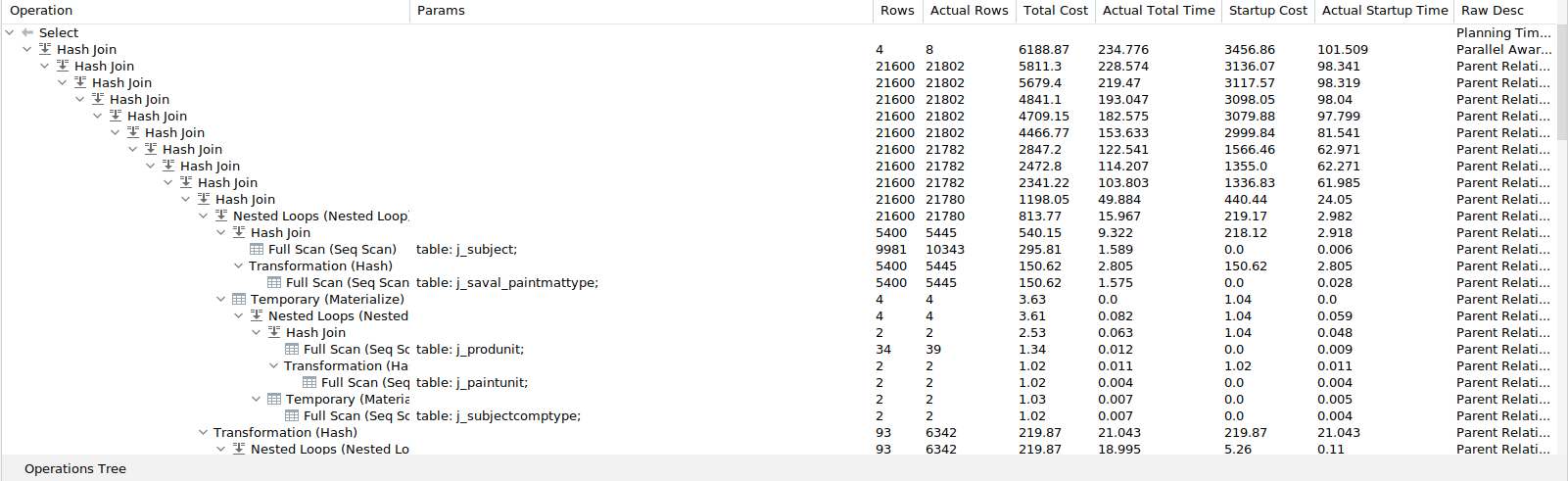If I put the constraint from the outer where clause directly into the subquery, like below, then it is fast (4ms). I get an extremely good plan with nested loops, where each step has at most ~300 rows:

``````select *
from subject as outer_subject
join (
SELECT inner_subject.id                   AS subject_id,
<more columns>
FROM   subject as inner_subject
JOIN <two pages query>
-- only the next line is added
WHERE  inner_subject.no in ('A31793357992','A2125336999S')
) csq
on csq.subject_id = outer_subject.id
where outer_subject.no in ('A31793357992','A2125336999S');
``````

With above version, I get this explain: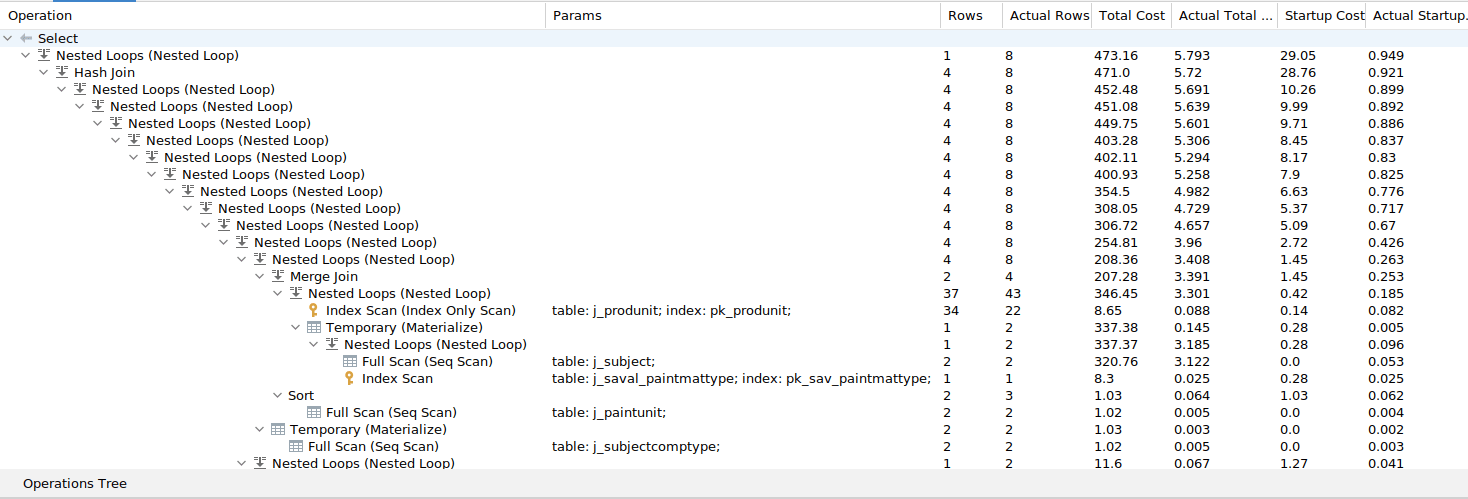I thought that the query optimizer should be able to propagate the constraint from the outer query into the subquery. But apparently this does not happen.

My question is, whether there is some fundamental thing preventing the optimizer from executing the first query as fast as the second one?

The other option is that the optimizer is just confused and accidentally does not find the good plan for the first query.

Any insights?

Apparently the DB performs a hash join in the first case, with a hash table of 20k rows and a loop of size 2.

Here is the relevant part of the execution plan (the table and column names might differ slightly form the examples above):

``````Hash Join  (cost=5251.03..5560.54 rows=4 width=54) (actual time=168.038..199.218 rows=8 loops=1)
Hash Cond: (f_t.sav_subject_id = s.s_id)
->  Hash Left Join  (cost=4930.24..5183.02 rows=21600 width=57) (actual time=162.068..194.481 rows=21646 loops=1)
<snip>
->  Hash  (cost=320.76..320.76 rows=2 width=18) (actual time=2.096..2.096 rows=2 loops=1)
Buckets: 1024  Batches: 1  Memory Usage: 9kB
->  Seq Scan on j_subject s  (cost=0.00..320.76 rows=2 width=18) (actual time=0.038..2.092 rows=2 loops=1)
Filter: ((s_no)::text = ANY ('{A31793357992,A2125336999S}'::text[]))
Rows Removed by Filter: 10292
Planning time: 21.553 ms
Execution time: 199.635 ms
``````

Postgres version:

PostgreSQL 10.5 on x86_64-pc-linux-gnu, compiled by gcc (GCC) 4.8.5 20150623 (Red Hat 4.8.5-28), 64-bit

Get this bounty!!!

## #StackBounty: #r #estimation #optimization #epidemiology #differential-equations SIR: parameter estimation and optimization here (R)

### Bounty: 100

From here https://ourworldindata.org/coronavirus/country/israel I have extracted the Covid Data for Israel, with some manipulations, I have obtained the plot of the daily new infections in Israel
If I want to create a SIR or SEIR model, e.g.

`````` dS <- -beta/N * I * S
dI <- beta/N * I * S - gamma * I
dR <- gamma * I
``````

but let’s say that beta can not be a constant over the whole time, but also a parameter, i.e $$beta = beta(tau)$$, $$beta$$ does not need to change every day it would be better if it stayed constant weekly or bi-weekly. (therefor I used $$tau$$ instaed of $$t$$). What would be efficient ways to estimate $$beta(tau)$$ from the number of daily new infections? (for sake of simplicity let gamma be constant over the whole time)

I have thought to somehow approximate the infection curve given below by polynomials or trigonometric functions, but I do not know what to after that? Because the functions would not be transmission rates at a given time $$tau$$. What do I have to do to obtain the transmission rates from the fitted piecewise functions, or should I use a whole other approach?

I would like to solve it in R. Ideally I would also like to somehow optimize the solution so that it more or less fits exactly to the data.

Any suggestions and help highly appreciated

(the data can be found here https://covid.ourworldindata.org/data/owid-covid-data.csv)

`````` covid <-  Covid[apply(Covid,1,function(x) {any(c("Israel") %in% x)}),]
covid[,1] <- NULL
covid[,2] <- NULL
covid <- as.data.frame(covid)
newcases <- diff(covid[,4])
``````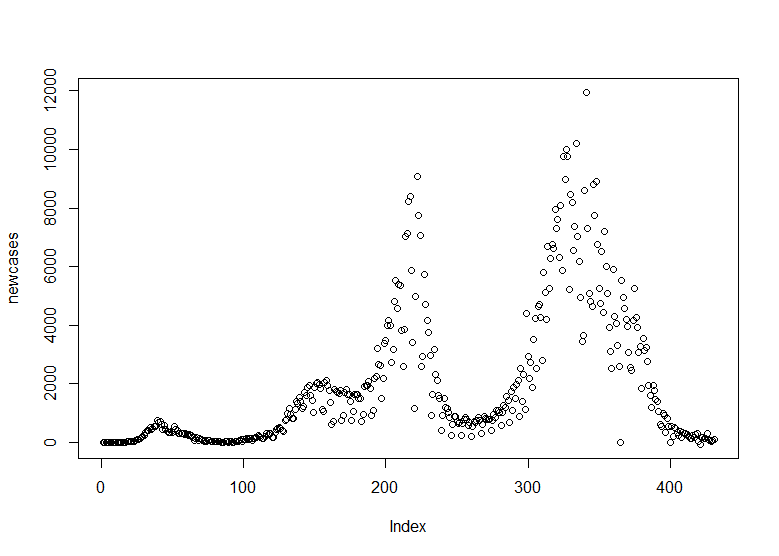Get this bounty!!!

## #StackBounty: #optimization #combinatorics Determining the number of maximum digits with coincidence restriction

### Bounty: 50

(I originally posted the question in math.stackexchange, but thought cross validated may be a better category for this problem)

Given set $$S$$ that contains numbers of base 3 that share the following characteristics:

• every element in $$S$$ share the same number of digits, $$x$$
• every element in $$S$$ can have leading zeros

We aim to solve the optimization problem $$max(x)$$, where when we select 6 elements from $$S$$, any randomly chosen pair of those 6 elements must have at most one coincidence.

(coincidence can be defined as a number of duplicate number in the same digit. 2010 and 1220, for instance, has a coincidence of one.)

I manually listed out the elements while increasing $$x$$ by 1, but soon realized that this is getting too tedious. I proved by trial and error that when $$x=4$$, the conditions match as $$S={0120, 1200, 2010, 0001, 1111, 2221}$$ works. I also suspect that when $$x=5$$, the conditions do not match (also done by trial and error), but I’d like to know if there is a mathematical way to confirm that.

I originally planned on using Pigeonhole Principle, but am quite unsure as to how to utilize it for the proof. Any insight is appreciated.

Get this bounty!!!

## #StackBounty: #optimization #association-rules #credit-scoring #tuning Streamlining / Optimizing conditional Rules in a rule engine usi…

### Bounty: 50

The problem is defined as follows :

We are dealing with a Rule engine used to classify a credit risk as ‘Good’, ‘Medium’ or ‘bad’. Say the rule engine which has say 10 rules. These rules are hierchical/ Reflexive in nature i.e. say :

Customer A comes in to apply for a product – he has to answer say 10 questions which could trigger those 10 rules if answered Yes. Say for e.g. the first question is :

1. Do u have any credit default in past ?
• Customer answers "Yes". This triggers say "default rule" and shoots the below question

1.1 : How long back did the default occur ?

• Action if time <2 years , trigger question 1.2 else Give decision "Good"
• Say customer answers 1 year and this triggers 1.2

1.2 How much was the default amount?

• Action if amount > 1000 dollars , trigger question 1.3 else Give decision "Medium"
• Say customer answers 5000 and this triggers 1.3 which is the final question in this rule

1.3 Was bankruptcy declared ?

• if Yes give decision "Bad"
• if No give decision "Bad"
• if in process , give decision "Bad"

Now the there could be different pathways for each customer based no their answers i.e. some just answer Q1.1 , Some go to Q1.1 & Q1.2 and some may go to Q1.1 , Q1.2 and Q1.3 and can get different decisions based on what they answer.

Problem statement is : Predicting the final decision – Good , Medium , Bad with minimal questions in this rule i.e. streamline if Q1.3 adds any value or not? for e.g. above we can stop at Q1.2 only where if default amount is > 1000 dollars , the outcome is always bad.

The problem is not trivial as in actual scenarios there are 100s of rules with long and multiple possible pathways. The idea is to streamline these rules (reduce the length) while achieving similar/ same level results performance. What possible approaches can be applied here if we build a model say for each rule to streamline it ?

So far my ideation :

1. Select top X rules with domain and volume considerations
2. Build model with various questions in pathway and their answers as variables and final outcome. Wherever question was not triggered , it will be NA.
3. See SHAP/additive value or information gain of questions where triggered to see if they add any value
4. Streamline and reduce (remove variables) based on 3.
5. rebuild model and compare accuracy with reduced variables(reduced questions)

One more consideration to keep in mind is Q1.3 is depending on Q1.2 and Q1.2 is on Q1.1 So we can’t reduce the question in between say Q1.2 and leave Q1.3 and Q1.1

Get this bounty!!!